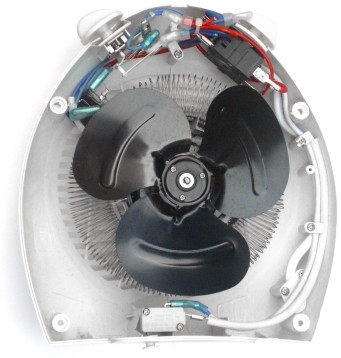# Electric PowerElectric power is the rate at which electrical energy is transferred.

The formula is Power = Current × Voltage:Power (P) is measured in Watts (W), Current (I) is measured in Amps (A), and Voltage (V) is measured in Volts (V).It takes both Current and Voltage to create Power!

This is similar to water where power needs both flow (like current) and pressure (like voltage).

See Electricity Water Analogy for more.

Let's use it:### Example: Charging a Phone

You are charging your smartphone using a USB charger. The charger provides a voltage of 5 V (Volts) and a current of 2.1 A (Amps).

P = IV
= 2.1 A × 5 V
= 10.5 W

So the power rate during charging is 10.5 W (Watts).

This tells us the rate that energy is being transferred, not the total amount of energy. For the total amount of energy we need to know how long the transfer took.Inside a Fan Heater

### Example: Fan Heater

The heater is connected to a 240 Volt power supply and draws a current of 8 Amp.

P = IV
= 8 A × 240 V
= 1920 W

#### Power

So the power consumed by the electric heater is 1920 W.

Which can be rounded to 2 kW (kiloWatts).

#### Energy

If we run the heater for 3 hours we have used:

Energy = 2 kW × 3 h = 6 kWh (kiloWatt hours) of electricity.

The energy is not lost to the world, it is converted to heat (and noise etc).

What if we are missing Current or Voltage but know Resistance instead?

Ohm's Law to the rescue:Ohm's Law

Combining P = IV with V = IR:

V = IR:P = I(IR)
Simplify:P = I2R
And also
Rearrange Ohm's Law so I = V/R:P = (V/R)V
Simplify:P = V2/R

So these are all valid ways to calculate electric power:

P = IV     P = I2R     P = V2/R### Example: Flashlight

The flashlight has two 1.5 V batteries and the light has a resistance of 6 Ω.

P = V2/R
= (1.5 + 1.5)2/6
= 32/6
= 1.5 W

## Power in General

Power is a general concept in Physics: it is energy flowing!

It is measured as energy per unit of time

• Energy is in Joules (J)
• Power is in Joules per second (J/s) which has the special unit of Watts (W)

1 Watt = 1 Joule of energy per second

1 W = 1 J/s

## Summary

• Power is the rate at which energy is transferred
• Power = Current × Voltage: P = IV
• Using Voltage = Current × Resistance (V = IR):
• P = I2R
• P = V2/R
• Energy = Power × Time